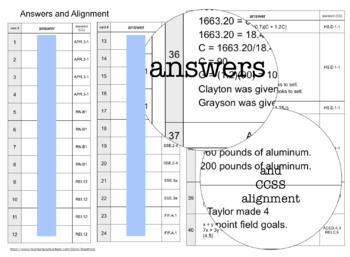# ALGEBRA 1 TEST PREP#1 - task cards (with paper version)8th - 11th
Subjects
Standards
Resource Type
Formats Included
• Zip
Pages
30+

#### Also included in

1. This bundle includes resources to make end of course review easier for you and your students.Use these for warm-ups, homework or assessments to determine student preparedness.Check out the individual products for more information!
\$13.75
\$16.20
Save \$2.45

### Description

ALGEBRA 1 TEST PREP

This resource also includes a paper version of the same problems for use as homework, intervention, test, etc.

Task cards are a popular math classroom resource, including test prep!
Teachers love task cards, and so do students!

These task cards are designed to be used for test prep and as such, include test-style questions. They are designed to mimic a testing format. Additionally, they can be used in any number of ways including; scavenger hunts, warm-ups/bell-ringers. exit tickets, and any task card activity (there are plenty of ideas - just google the term, “task cards”

The skills in this set were chosen to prepare for testing that is typically done in the first of three sessions or testing windows. However, they can be used for any curriculum design.

These cards cover skills assessed in a first unit, including;

Operations with polynomial (APR.1)
Properties of rational and irrational numbers (RN.3)
Representing solutions graphically (REI.10)
Solving equations and inequalities graphically (REI.12)
Using the structure of expressions (SSE.2)
Writing expressions in equivalent forms (SSE.3)
Using function notation and the concept of a function(FIF.1)
Interpreting functions in context (FIF.4)
Analyzing and comparing two functions (FIF.9)
Creating equations that describe relationships (ACED.3)
Identifying the effect of a value k on a function (FBF.3)
Solving systems of equations and inequalities (REI.6)

included are

40 TEST PREP task cards for Algebra 1
Paper version of the 40 questions
Alignment (CCSS)

Consider the other sets in this series; combined they give you 120 questions to prepare your students!

ALG1 Test Prep cards set TWO

ALG1 Test Prep cards set THREE
Total Pages
30+
N/A
Teaching Duration
N/A
Report this Resource to TpT
Reported resources will be reviewed by our team. Report this resource to let us know if this resource violates TpT’s content guidelines.

### Standards

to see state-specific standards (only available in the US).
Identify the effect on the graph of replacing 𝘧(𝘹) by 𝘧(𝘹) + 𝘬, 𝘬 𝘧(𝘹), 𝘧(𝘬𝘹), and 𝘧(𝘹 + 𝘬) for specific values of 𝘬 (both positive and negative); find the value of 𝘬 given the graphs. Experiment with cases and illustrate an explanation of the effects on the graph using technology.
Compare properties of two functions each represented in a different way (algebraically, graphically, numerically in tables, or by verbal descriptions). For example, given a graph of one quadratic function and an algebraic expression for another, say which has the larger maximum.
For a function that models a relationship between two quantities, interpret key features of graphs and tables in terms of the quantities, and sketch graphs showing key features given a verbal description of the relationship.
Understand that a function from one set (called the domain) to another set (called the range) assigns to each element of the domain exactly one element of the range. If 𝘧 is a function and 𝘹 is an element of its domain, then 𝘧(𝘹) denotes the output of 𝘧 corresponding to the input 𝘹. The graph of 𝘧 is the graph of the equation 𝘺 = 𝘧(𝘹).
Graph the solutions to a linear inequality in two variables as a half-plane (excluding the boundary in the case of a strict inequality), and graph the solution set to a system of linear inequalities in two variables as the intersection of the corresponding half-planes.